# Algebra II : Circle Functions

## Example Questions

2 Next →

### Example Question #11 : Circle Functions

Determine the center and radius, respectively, given the equation: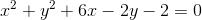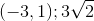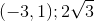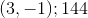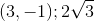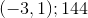Explanation:

In order to solve for the radius, we will need to complete the square twice.

Group the x and y-variables in parentheses.  Starting from the original equation: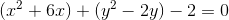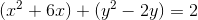Divide by the second term coefficient of each binomial by 2, and add the squared quantity on both sides of the equation.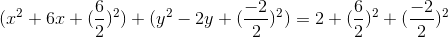The equation becomes: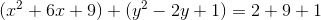Factorize both polynomials in parentheses and simplify the right side.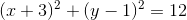The center is: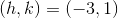The radius is:The answer is:2 Next →

### All Algebra II Resources